• 基于常规蚁群算法进行了数学模型的构建、算法结构分析，并采用残留信息素数量限制、信息素的持久性系数自适应控制和全局更新规则对算法进行了加强设计，提出了双参数交叉影响的连续域组合优化蚁群算法；同时通过选取...连续优化问题
• 论文研究-基于改进蚁群算法设计的敏捷卫星调度方法.pdf, 敏捷卫星与传统非敏捷卫星相比，增加了俯仰和偏航两个自由度，提升了卫星的成像能力，也加大了搜索空间，使敏捷...
• 蚁群算法(ant colony optimization, ACO)，又称...针对PID控制器参数优化设计问题,将蚁群算法设计的结果与遗传算法设计的结果进行了比较,数值仿真结果表明,蚁群算法具有一种新的模拟进化优化方法的有效性和应用价值。
•文档
• 面向QoS路由问题，设计了一种基于遗传算法和蚁群算法融合的QoS路由算法（QoS routing algorithm according to the combination of the genetic algorithm and ant colony algorithm，GAACO_QoS）。利用遗传算法生成...遗传算法
• 利用该框架实现了解决TSP问题的蚁群算法，给出了程序实现的设计思想和框架结构，描述了运行过程，并分析了蚁群算法的主要参数对算法结果的影响，说明Repast不仅是一个高效的用于多Agent仿真开发工具，也适用于类似...
• 通过对拉式膜片弹簧载荷一变形特性的综合分析,考虑各种约束条件,提出了一种新的多目标优化设计数学模型....将遗传算法和蚁群算法结合起来,在蚁群算法的每一次迭代中,首先根据信息量选择解分量的初值,然后使用变异操作
• 基于此，研究了附带翻转工位操作的挖掘机底盘双边装配线规划设计问题，针对该问题提出了一种改进蚁群算法求解。给出了问题求解的启发式任务分配规则，提出可采用启发式任务选择规则以提高算法收敛速率。进而分析某型...
• 1.蚁群算法简介 蚁群算法（Ant Clony Optimization， ACO）是一种群智能算法，它是由一群无智能或有轻微智能的个体（Agent）通过相互协作而表现...针对PID控制器参数优化设计问题，将蚁群算法设计的结果与遗传算法设计
1.蚁群算法简介
蚁群算法（Ant Clony Optimization， ACO）是一种群智能算法，它是由一群无智能或有轻微智能的个体（Agent）通过相互协作而表现出智能行为，从而为求解复杂问题提供了一个新的可能性。蚁群算法是一种用来寻找优化路径的概率型算法。它由Marco Dorigo于1992年在他的博士论文中提出，其灵感来源于蚂蚁在寻找食物过程中发现路径的行为。蚁群算法是一种模拟进化算法，初步的研究表明该算法具有许多优良的性质。针对PID控制器参数优化设计问题，将蚁群算法设计的结果与遗传算法设计的结果进行了比较，数值仿真结果表明，蚁群算法具有一种新的模拟进化优化方法的有效性和应用价值。 蚁群算法是一种仿生学算法，是由自然界中蚂蚁觅食的行为而启发的。在自然界中，蚂蚁觅食过程中，蚁群总能够按照寻找到一条从蚁巢和食物源的最优路径。图（1）显示了这样一个觅食的过程。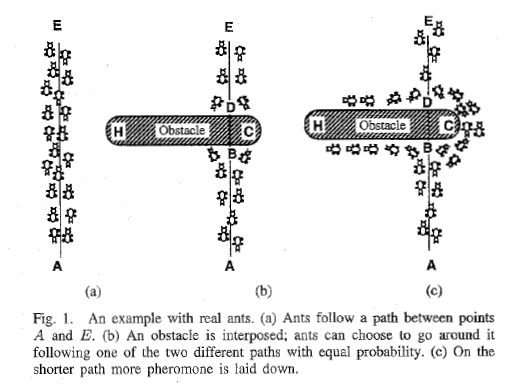在图1（a）中，有一群蚂蚁，假如A是蚁巢，E是食物源（反之亦然）。这群蚂蚁将沿着蚁巢和食物源之间的直线路径行驶。假如在A和E之间突然出现了一个障碍物（图1（b）），那么，在B点（或D点）的蚂蚁将要做出决策，到底是向左行驶还是向右行驶？由于一开始路上没有前面蚂蚁留下的信息素（pheromone），蚂蚁朝着两个方向行进的概率是相等的。但是当有蚂蚁走过时，它将会在它行进的路上释放出信息素，并且这种信息素会以一定的速率散发掉。信息素是蚂蚁之间交流的工具之一。它后面的蚂蚁通过路上信息素的浓度，做出决策，往左还是往右。很明显，沿着短边的的路径上信息素将会越来越浓（图1（c）），从而吸引了越来越多的蚂蚁沿着这条路径行驶。
2.TSP问题描述
蚁群算法最早用来求解TSP问题，并且表现出了很大的优越性，因为它分布式特性，鲁棒性强并且容易与其它算法结合，但是同时也存在这收敛速度慢，容易陷入局部最优（local optimal）等缺点。 TSP问题（Travel Salesperson Problem，即旅行商问题或者称为中国邮递员问题），是一种NP-hard问题，此类问题用一般的算法是很大得到最优解的，所以一般需要借助一些启发式算法求解，例如遗传算法（GA），蚁群算法（ACO），微粒群算法（PSO）等等。 TSP问题可以分为两类，一类是对称TSP问题（Symmetric TSP），另一类是非对称问题（Asymmetric TSP）。所有的TSP问题都可以用一个图（Graph）来描述： 令V={C1,C2,…,Ci,…,Cn},i=1,2,…,n是所有城市的集合，Ci表示第i个城市，n为城市的数目； E={(r,s):r,s∈V}是所有城市之间连接的集合； C={Crs:r,s∈V}是所有城市之间连接的成本度量（一般为城市之间的距离）； 如果Crs=Csr，那么该TSP问题为对称的，否则为非对称的。 一个TSP问题可以表达为： 求解遍历图G=(V,E,C)，所有的节点一次并且回到起始节点，使得连接这些节点的路径成本最低。
3.蚁群算法原理
假如蚁群中所有蚂蚁的数量为m，所有城市之间的信息素用矩阵pheromone表示，最短路径为bestLength，最佳路径为bestTour。每只蚂蚁都有自己的内存，内存中用一个禁忌表（Tabu）来存储该蚂蚁已经访问过的城市，表示其在以后的搜索中将不能访问这些城市；还有用另外一个允许访问的城市表（Allowed）来存储它还可以访问的城市；另外还用一个矩阵（Delta）来存储它在一个循环（或者迭代）中给所经过的路径释放的信息素；还有另外一些数据，例如一些控制参数(α，β，ρ，Q)，该蚂蚁行走完全程的总成本或距离（tourLength），等等。假定算法总共运行MAX_GEN次，运行时间为t。 蚁群算法计算过程如下： （1）初始化 设t=0，初始化bestLength为一个非常大的数（正无穷），bestTour为空。初始化所有的蚂蚁的Delt矩阵所有元素初始化为0，Tabu表清空，Allowed表中加入所有的城市节点。随机选择它们的起始位置（也可以人工指定）。在Tabu中加入起始节点，Allowed中去掉该起始节点。 （2）为每只蚂蚁选择下一个节点。 为每只蚂蚁选择下一个节点，该节点只能从Allowed中以某种概率（公式1）搜索到，每搜到一个，就将该节点加入到Tabu中，并且从Allowed中删除该节点。该过程重复n-1次，直到所有的城市都遍历过一次。遍历完所有节点后，将起始节点加入到Tabu中。此时Tabu表元素数量为n+1（n为城市数量），Allowed元素数量为0。接下来按照（公式2）计算每个蚂蚁的Delta矩阵值。最后计算最佳路径，比较每个蚂蚁的路径成本，然后和bestLength比较，若它的路径成本比bestLength小，则将该值赋予bestLength，并且将其Tabu赋予BestTour。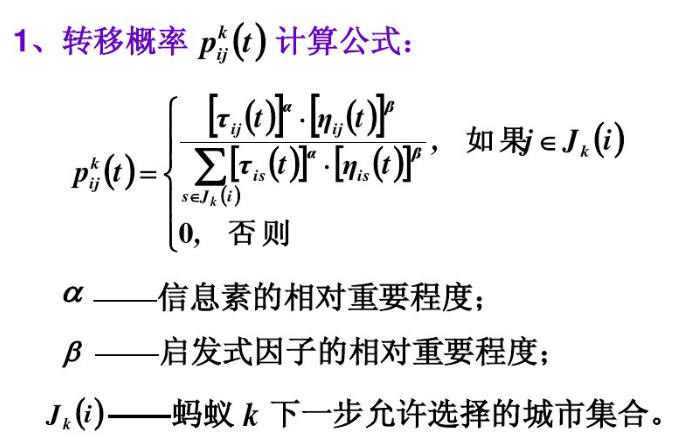（公式1）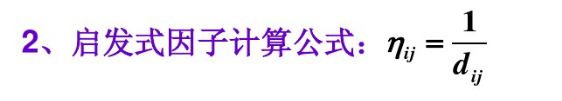（公式2）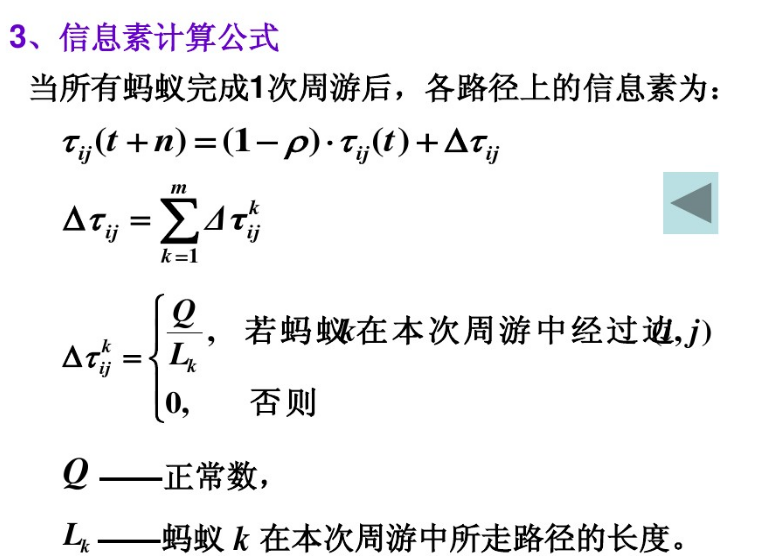（公式3）
其中pij(t)表示选择城市j的概率，k表示第k个蚂蚁，τij (t)表示城市i,j在第t时刻的信息素浓度，ηij表示从城市i到城市j的可见度，ηij=1/dij，dij表示城市i,j之间的成本（或距离）。由此可见dij越小，ηij越大，也就是从城市i到j的可见性就越大。 Δτkij表示蚂蚁k在城市i与j之间留下的信息素。 Lk表示蚂蚁k经过一个循环（或迭代）锁经过路径的总成本（或距离），即tourLength,α,β,Q 均为控制参数。 （3）更新信息素矩阵 令t=t+nt，按照（公式3）更新信息素矩阵phermone。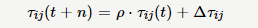（公式4）
τij(t+n)为t+n时刻城市i与j之间的信息素浓度。ρ为控制参数，Deltaij为城市i与j之间信息素经过一个迭代后的增量。并且有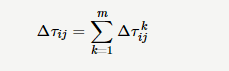（公式5）
其中Δτkij由公式计算得到。 （4）检查终止条件 如果达到最大代数MAX_GEN，算法终止，转到第（5）步；否则，重新初始化所有的蚂蚁的Delt矩阵所有元素初始化为0，Tabu表清空，Allowed表中加入所有的城市节点。随机选择它们的起始位置（也可以人工指定）。在Tabu中加入起始节点，Allowed中去掉该起始节点，重复执行（2），（3）,(4)步。 （5）输出最优值
4.算法流程图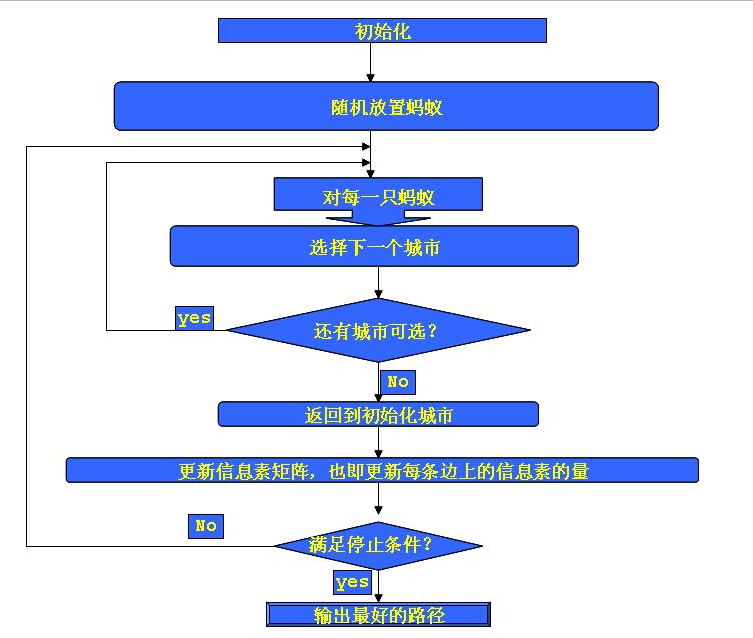5.java实现
在该java实现中我们选择使用tsplib上的数据att48，这是一个对称tsp问题，城市规模为48，其最优值为10628.其距离计算方法如图（2）所示：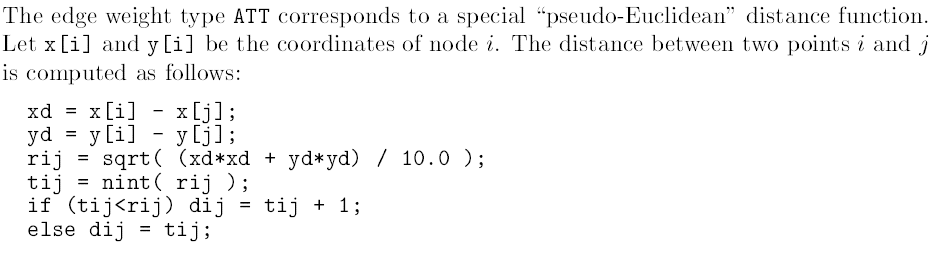图2 att48距离计算方法
实现中，使用了两个java类，一个Ant类，一个ACO类。 Ant类
package com.gbs.bean;

import java.util.ArrayList;
import java.util.Arrays;
import java.util.Collection;
import java.util.Collections;
import java.util.Random;
/**
* 蚂蚁类
* Cloneable这个类为克隆类，使用Object的clone()方法
* @author gbs
*
*/
public class Ant implements Cloneable{

/**
* Vector与ArrayList一样，也是通过数组实现的，不同的是它支持线程的同步，
* 即某一时刻只有一个线程能够写Vector，避免多线程同时写而引起的不一致性，
* 但实现同步需要很高的花费，因此，访问它比访问ArrayList慢。
*/
private ArrayList<Integer> allowedCities;//允许访问的城市

private ArrayList<Integer> tabu;//禁忌表，记录已经访问过的城市

private int [][] distance;//距离矩阵

private double[][] delta; //信息素变化矩阵

private double alpha;

private double beta;

private int cityNum;//城市数量

private int tourLength;//路径的长度

private int firstCity; //起始城市

private int currentCity; //当前城市

public Ant(int cityNum) {
this.cityNum = cityNum;
this.tourLength = 0;
}

public Ant() {
this.cityNum = 30;
this.tourLength = 0;
}

/**
* 初始化蚂蚁，并为蚂蚁随机选择第一个城市
* @param distance
* @param a
* @param b
*/
public void init(int[][] distance,double a,double b) {
this.alpha = a;
this.beta = b;
this.distance = distance;
this.allowedCities = new ArrayList<Integer>();
this.tabu = new ArrayList<Integer>();
this.delta = new double[cityNum][cityNum];
for(int i = 0;i < this.cityNum;i++) {
Integer city = new Integer(i);
for (int j = 0;j < this.cityNum;j++) {
this.delta[i][j] = 0.d;
}
}

Random random = new Random(System.currentTimeMillis());
this.firstCity = random.nextInt(this.cityNum);

for (Integer city:this.allowedCities) {
if(city.intValue() == this.firstCity) {
this.allowedCities.remove(city);
break;
}
}

this.currentCity = this.firstCity;
}

/**
* 选择下一个城市
* @param pheromone 信息素矩阵
*/
public void selectNextCity(double[][] pheromone) {
double[] p = new double[this.cityNum];//转移概率
double sum = 0.d;//转移概率的分母
for (Integer city : this.allowedCities) {
sum += Math.pow(pheromone[this.currentCity][city.intValue()],
this.alpha)*Math.pow(1.d/this.distance[this.currentCity][city.intValue()], this.beta);
}
for (int i = 0; i < this.cityNum; i++) {
boolean flag = false;
for (Integer city : this.allowedCities) {
if(i == city.intValue()) {
p[i] = (double) ((Math.pow(pheromone[this.currentCity][i],
this.alpha)*Math.pow(1.d/this.distance[this.currentCity][i], this.beta))/sum);
flag = true;
break;
}
}
if(!flag)
p[i] = 0.d;
}

/**
* 如果每次都直接选择最大概率的城市作为下一个城市，就会使算法过早收敛，
* 最后停止搜索可能获得的仅仅是次优解，而使用轮盘赌可以提高算法的全局搜索能力，又不失局部搜索
* 所以这里选择轮盘赌选择下一个城市。参考《计算智能》清华大学出版社
*/
//轮盘赌选择下一个城市
double sumSelect = 0.d;
int selectCity = -1;
Random random = new Random(System.currentTimeMillis());
double selectP = random.nextDouble();
while(selectP == 0.f) {
selectP = random.nextDouble();
}
for(int i = 0;i < this.cityNum;i++) {
sumSelect += p[i];
if(sumSelect >= selectP) {
selectCity = i;
//从允许选择的城市中去除select city
this.allowedCities.remove(Integer.valueOf(selectCity));
//在禁忌表中添加select city
//将当前城市改为选择的城市
this.currentCity = selectCity;
break;
}
}
}

/**
* 计算路径长度
* @return
*/
public int calculateTourLength() {
int length = 0;

for(int i = 0;i < this.tabu.size()-1;i++) {
length += this.distance[this.tabu.get(i).intValue()][this.tabu.get(i+1).intValue()];
}
return length;
}

public ArrayList<Integer> getAllowedCities() {
return allowedCities;
}

public void setAllowedCities(ArrayList<Integer> allowedCities) {
this.allowedCities = allowedCities;
}

public ArrayList<Integer> getTabu() {
return tabu;
}

public void setTabu(ArrayList<Integer> tabu) {
this.tabu = tabu;
}

public int[][] getDistance() {
return distance;
}

public void setDistance(int[][] distance) {
this.distance = distance;
}

public double[][] getDelta() {
return delta;
}

public void setDelta(double[][] delta) {
this.delta = delta;
}

public double getAlpha() {
return alpha;
}

public void setAlpha(double alpha) {
this.alpha = alpha;
}

public double getBeta() {
return beta;
}

public void setBeta(double beta) {
this.beta = beta;
}

public int getCityNum() {
return cityNum;
}

public void setCityNum(int cityNum) {
this.cityNum = cityNum;
}

public int getTourLength() {
}

public void setTourLength(int tourLength) {
this.tourLength = tourLength;
}

public int getFirstCity() {
return firstCity;
}

public void setFirstCity(int firstCity) {
this.firstCity = firstCity;
}

public int getCurrentCity() {
return currentCity;
}

public void setCurrentCity(int currentCity) {
this.currentCity = currentCity;
}

@Override
public String toString() {
return "Ant [allowedCities=" + allowedCities + ", tabu=" + tabu + ", distance=" + Arrays.toString(distance)
+ ", delta=" + Arrays.toString(delta) + ", alpha=" + alpha + ", beta=" + beta + ", cityNum=" + cityNum
+ ", tourLength=" + tourLength + ", firstCity=" + firstCity + ", currentCity=" + currentCity + "]";
}

}
ACO类
package com.gbs.bean;

import java.io.FileInputStream;
import java.io.FileNotFoundException;
import java.io.IOException;
import java.util.Arrays;

/**
* 蚁群算法类
* @author gbs
*
*/
public class ACO {

private Ant[] ants; //蚂蚁
private int antNum; //蚂蚁数量
private int cityNum; //城市数量
private int MAX_GEN; //运行代数
private double[][] pheromone; //信息素矩阵
private int[][] distance; //距离矩阵
private int bestLength; //最佳长度
private int[] bestTour; //最佳路径
//三个参数
private double alpha;
private double beta;
private double rho;

public ACO() {

}

public ACO(int antNum, int cityNum, int mAX_GEN, double alpha, double beta, double rho) {
this.antNum = antNum;
this.cityNum = cityNum;
this.MAX_GEN = mAX_GEN;
this.alpha = alpha;
this.beta = beta;
this.rho = rho;
this.ants = new Ant[this.antNum];
}

public void init(String path) {
int []x;
int []y;
String buffer;
try {
this.distance = new int[this.cityNum][this.cityNum];
x = new int[cityNum];
y = new int[cityNum];
//读取城市的坐标
for (int i = 0; i < cityNum; i++) {
String[] str = buffer.split(" ");
x[i] = Integer.valueOf(str);
y[i] = Integer.valueOf(str);
}
/**
* 计算距离矩阵 ，针对具体问题，距离计算方法也不一样，此处用的是att48作为案例，
* 它有48个城市，距离计算方法为伪欧氏距离，最优值为10628
*/
for(int i = 0;i < this.cityNum - 1;i++) {
for(int j = i + 1;j < this.cityNum;j++) {
double rij = Math.sqrt(((x[i]-x[j])*(x[i]-x[j]) + (y[i]-y[j])*(y[i]-y[j]))/10.0);
int tij = (int)Math.round(rij);
if(tij < rij)
tij++;
this.distance[i][j] = tij;
this.distance[j][i] = tij;
}
}
this.distance[this.cityNum-1][this.cityNum-1] = 0;
//初始化信息素矩阵
this.pheromone=new double[this.cityNum][this.cityNum];
for(int i = 0;i < this.cityNum;i++) {
for(int j = 0;j < this.cityNum;j++) {
this.pheromone[i][j] = 0.1d;
}
}
//初始化最优路径的长度
this.bestLength=Integer.MAX_VALUE;
//初始化最优路径
this.bestTour=new int[this.cityNum+1];
//随机放置蚂蚁
for(int i = 0;i < this.antNum;i++){
this.ants[i]=new Ant(this.cityNum);
this.ants[i].init(this.distance, this.alpha, this.beta);
}
} catch (FileNotFoundException e) {
e.printStackTrace();
} catch (IOException e) {
e.printStackTrace();
}
}

/**
* 更新信息素
*/
private void updatePheromone() {
//信息素挥发
for(int i = 0;i < this.cityNum;i++)
for(int j = 0;j < this.cityNum;j++)
this.pheromone[i][j] = this.pheromone[i][j] * (1 - this.rho);
//信息素更新
for(int i = 0;i < this.cityNum;i++) {
for(int j = 0;j < this.cityNum;j++) {
for(int k = 0;k < this.antNum;k++) {
this.pheromone[i][j] += this.ants[k].getDelta()[i][j];
}
}
}
}

public void solve() {
for (int g = 0; g < this.MAX_GEN; g++) {
//每一只蚂蚁移动的过程
for (int i = 0; i < this.antNum; i++) {
for (int j = 0; j < this.cityNum; j++) {
this.ants[i].selectNextCity(this.pheromone);
}
//计算蚂蚁获得的路径长度
this.ants[i].setTourLength(this.ants[i].calculateTourLength());
if(this.ants[i].getTourLength() < this.bestLength){
//保留最优路径
this.bestLength = this.ants[i].getTourLength();
System.out.println("第"+g+"代，发现新的解"+this.bestLength);
for(int k = 0;k < this.ants[i].getTabu().size();k++)
this.bestTour[k] = this.ants[i].getTabu().get(k).intValue();;
}
//更新信息素变化矩阵
for (int j = 0; j < this.ants[i].getTabu().size()-1; j++) {
this.ants[i].getDelta()[this.ants[i].getTabu().get(j).intValue()][this.ants[i].getTabu().get(j+1).intValue()] = (double) (1.0/this.ants[i].getTourLength());
this.ants[i].getDelta()[this.ants[i].getTabu().get(j+1).intValue()][this.ants[i].getTabu().get(j).intValue()] = (double) (1.0/this.ants[i].getTourLength());
}
}
//更新信息素
this.updatePheromone();
//重新初始化蚂蚁
for(int i = 0;i < this.antNum;i++){
this.ants[i].init(this.distance, this.alpha, this.beta);
}
}
//打印最佳结果
this.printOptimal();
}

public void printOptimal() {
System.out.println("The optimal length is: " + this.bestLength);
System.out.println("The optimal tour is: ");
for (int i = 0; i < this.bestTour.length; i++) {
System.out.println(this.bestTour[i]);
}
}

public Ant[] getAnts() {
return ants;
}

public void setAnts(Ant[] ants) {
this.ants = ants;
}

public int getAntNum() {
return antNum;
}

public void setAntNum(int antNum) {
this.antNum = antNum;
}

public int getCityNum() {
return cityNum;
}

public void setCityNum(int cityNum) {
this.cityNum = cityNum;
}

public int getMAX_GEN() {
return MAX_GEN;
}

public void setMAX_GEN(int mAX_GEN) {
MAX_GEN = mAX_GEN;
}

public double[][] getPheromone() {
return pheromone;
}

public void setPheromone(double[][] pheromone) {
this.pheromone = pheromone;
}

public int[][] getDistance() {
return distance;
}

public void setDistance(int[][] distance) {
this.distance = distance;
}

public int getBestLength() {
return bestLength;
}

public void setBestLength(int bestLength) {
this.bestLength = bestLength;
}

public int[] getBestTour() {
return bestTour;
}

public void setBestTour(int[] bestTour) {
this.bestTour = bestTour;
}

public double getAlpha() {
return alpha;
}

public void setAlpha(double alpha) {
this.alpha = alpha;
}

public double getBeta() {
return beta;
}

public void setBeta(double beta) {
this.beta = beta;
}

public double getRho() {
return rho;
}

public void setRho(double rho) {
this.rho = rho;
}

@Override
public String toString() {
return "ACO [ants=" + Arrays.toString(ants) + ", antNum=" + antNum + ", cityNum=" + cityNum + ", MAX_GEN="
+ MAX_GEN + ", pheromone=" + Arrays.toString(pheromone) + ", distance=" + Arrays.toString(distance)
+ ", bestLength=" + bestLength + ", bestTour=" + Arrays.toString(bestTour) + ", alpha=" + alpha
+ ", beta=" + beta + ", rho=" + rho + "]";
}

}
Test类：
package com.gbs.test;

import com.gbs.bean.ACO;

public class Test {
public static void main(String[] args) {
ACO aco = new ACO(200, 48, 2000, 1.d, 5.d, 0.5d);
aco.init("d://cities.txt");
aco.solve();
}
}
注：调试代码时遇到一个致命的问题就是忽略了float和double的精度。浪费了三四个小时debug居然是这个错误引起的，float的精度一旦不够，在java中会出现NAN和INFINITY。https://www.cnblogs.com/zhisuoyu/archive/2016/03/24/5314541.html（此网址讲述了NAN和INFINITY的区别）。
6.存在问题
（1）对于大规模组合优化问题，算法的计算时间而且复杂。由于蚁群算法的时间复杂度是，因此在处理较大规模的组合优化问题时，运算量较大，时间较长。 （2）算法容易在某个或某些局部最优解的邻域附近发生停滞现象，造成早熟收敛，即搜索进行到一定程度后，所有蚂蚁发现的解完全一致，不能继续对解空间进一步搜索，不利于发现全局最优解。 （3）不能较好的解决连续域问题。 （4）由于蚁群算法中蚂蚁个体的运动过程的随机性，当群体规模设置较大时，很难在较短时间内从杂乱无章的路径中找出一条较好的路径。 （5）信息素更新策略，路径搜索策略和最优解保留策略都带有经验性，没有经过严格的理论论证。因此基本蚁群算法的求解效率不高、收敛性较差、求解结果具有较大的分散性。

展开全文• 蚁群算法(ant colony optimization, ACO)，又称...针对PID控制器参数优化设计问题，将蚁群算法设计的结果与遗传算法设计的结果进行了比较，数值仿真结果表明，蚁群算法具有一种新的模拟进化优化方法的有效性和应用价值
• 描述了Job-shop调度问题，研究遗传算法和蚁群算法在解决Job-shop问题中的优点和不足，融合遗传算法和蚁群算法设计了遗传蚁群算法以求解Job-shop调度问题，并对算法进行了仿真实验，通过与遗传算法、蚁群算法及已有的...遗传算法
• 蚁群算法(ant colony optimization, ACO)，又称...针对PID控制器参数优化设计问题,将蚁群算法设计的结果与遗传算法设计的结果进行了比较,数值仿真结果表明,蚁群算法具有一种新的模拟进化优化方法的有效性和应用价值。
• 蚁群算法(ant colony optimization, ACO)，又...针对PID控制器参数优化设计问题，将蚁群算法设计的结果与遗传算法设计的结果进行了比较，数值仿真结果表明，蚁群算法具有一种新的模拟进化优化方法的有效性和应用价值。
• 针对如何提高蚁群算法搜索速度及防止算法停滞问题,提出一种改进的蚁群优化算法 VACO( ACO algorithm based on ant velocity),通过构造与局部路径和蚂蚁个体速度相关的时间函数,并建立与时间函数相关的动态信息素 ...
• 针对PID控制器参数优化设计问题，将蚁群算法设计的结果与遗传算法设计的结果进行了比较，数值仿真结果表明，蚁群算法具有一种新的模拟进化优化方法的有效性和应用价值。 定义 各个蚂蚁在没有事先告诉他们食物在什么...
• 为了增强无线传感器网络的动态适应性和实现数据包的多路径传输, 根据蚁群算法的原理, 使用NesC语言在TinyOS2. x下设计了路由协议Ant-PDRP。该协议采用信息素浓度指引路由包和数据包传输, 并在数据包传输过程中引入...无线传感器网络 路由协议
• 该算法改变蚁群算法中信息素的释放方式，采用量子优化算法中的量子概率幅代替，信息素的更新则通过使用量子相位旋转的方式，实现蚂蚁信息素的自适应更新，用于有效地降低蚁群算法容易早熟收敛的情况。通过实验对比...片上网络 低功耗
• 利用蚁群算法的基本原理，将多维有约束的优化问题通过罚惩因子方式转换为统一的多变量目标函数形式，并将所有独立变量分成不同的等份区域，以蚂蚁走过每一变量的一个区域并访问完所有变量所构成的构造图作为优化问题...多维函数优化
• 论文研究-求解复杂TSP问题的随机扰动蚁群算法.pdf, 针对基本蚁群算法 ,设计出一种新颖的随机扰动蚁群算法 ,并将其应用于求解复杂 TSP问题 .该算法包含了两个重要方面 :...
• 量子蚁群算法的小论文，详细介绍了MapReduce架构及算法设计步骤
• 论文研究-基于蚁群算法的拣选作业优化问题.pdf, 蚁群算法是一种新型的启发式算法,研究表明该算法具有较强发现较好解的能力,但同时存在一些缺点如易出现停滞现象、 收敛...
• 在SimuroSot5v5的中场策略中引入蚁群算法，通过采用信息素分区获取的方式建立起球队中场队形转换机制。该机制以球的位置为驱动信息，根据求取的信息素来确定出适当的中场队形；同时设计任务死锁的自调节模块来提高...机器人足球
• 蚁群算法(ant colony optimization, ACO)，又...针对PID控制器参数优化设计问题，将蚁群算法设计的结果与遗传算法设计的结果进行了比较，数值仿真结果表明，蚁群算法具有一种新的模拟进化优化方法的有效性和应用价值。
• 量子蚁群算法的小论文设计新的思路求解传统TSP问题，并对结果进行了验证对比
• 研究了多时间窗车辆路径问题，建立了多时间窗车辆路径问题的数学模型，并基于蚁群算法设计了一种混合蚁群算法对问题进行了求解。该算法首先利用基本蚁群算法求解，然后采用2-opt算法和元胞自动算法对结果进行优化，......